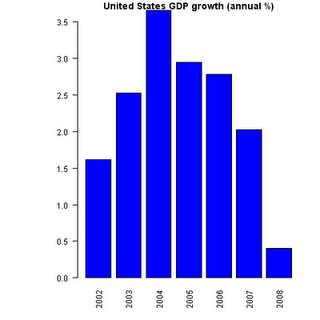Want to share your content on R-bloggers? click here if you have a blog, or here if you don't.

Copy and paste the code listed in this post below, and you can create a plot of World Bank data using R with a call like the following:

plotWorldBank(‘USA’,’NY.GDP.MKTP.KD.ZG’)There is a wealth of economic and demographic available at the World Bank. They are also interested in developers using their data to produce applications. You can see their site for a full description and terms of use for their APIs. The following R function produces a simple bar plot of indicator data for a selected time period for a given country (and produced the chart above).

library(‘XML’)

plotWorldBank= function (country=’US’,
indicator=’NY.GDP.MKTP.CD’,
start_year=2002,
end_year=2008,
color=’blue’
){
# Construct the URL
url = paste(‘http://open.worldbank.org/countries/’,
country,
‘/indicators/’,
indicator,
‘?date=’,
start_year,
‘:’,
end_year,
sep=”)

# Print URL for reference
print(url)

# Parse the XML
doc = xmlTreeParse(url, useInternal = TRUE)

# Extract the relevant values
indicator = xmlValue(getNodeSet(doc, “//wb:indicator”)[])
countryName = xmlValue(getNodeSet(doc, “//wb:country “)[])
values = sapply(getNodeSet(doc, “//wb:value”) , function(el) xmlValue(el))
dates = sapply(getNodeSet(doc, “//wb:date”) , function(el) xmlValue(el))
names(values)=dates

# Plot the data
par(las=2,mar=c(4, 8, 1, 2) + 0.1)
barplot(t(rev(values)), main=paste(countryName ,indicator),
col=color)
}

Parameter Usage

The parameter values must be in the format specified by the world bank API. A few examples of these three character codes:

AUS Australia
BMU Bermuda
CHE Switzerland
GBR United Kingdom
USA United States

The list of county codes(in XML) can be found on pages starting here.

Likewise, special indicator codes are used to select the data set in question. These indicators cover quite a large variety of subjects including economic, energy, environmental, and social interests.

A few examples:

NY.GDP.MKTP.CD GDP (current US\$)
NY.GDP.MKTP.KD.ZG GDP growth (annual %)
AG.CON.FERT.MT Fertilizer consumption (metric tons)
SH.XPD.TOTL.ZS Health expenditure, total (% of GDP)
ST.INT.ARVL International tourism, number of arrivals

The list of indicators (in XML) can be found here.